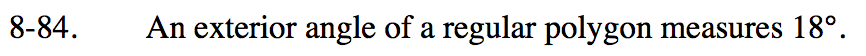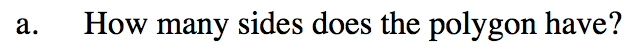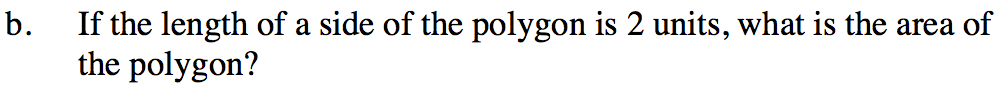Home > INT2 > Chapter 8 > Lesson 8.4.1 > Problem8-84

8-84.
1. An exterior angle of a regular polygon measures 18°. Homework Help ✎

1. How many sides does the polygon have?

2. If the length of a side of the polygon is 2 units, what is the area of the polygon?Remember that the sum of the exterior angles is 360°.Refer to the Math Notes box in Lesson 8.4.1, which gives a step-by-step
example illustrating how to solve this type of problem.

≈ 126.3 units2